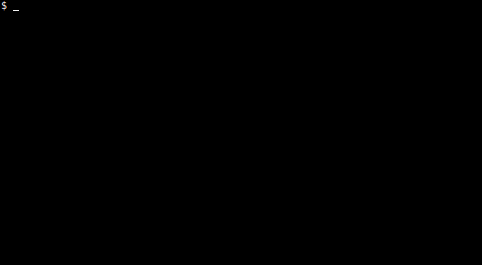```             /*at the Olympic opening ceremony in Seoul*/
/*, a flock of doves flew into the big*/
/*cauldron. their wings melted to orange*/
/*like rings interlocked with rings.*/
/*they're betting all over the world*/
/*on when the cloud chamber will win*/
/*the lottery, free from faint astrology.*/
/*celestial bodies roll around the heavens*/
/*with the confirmation bias of a bowl.*/
/*you dream about the way the wind blew*/
/*that day as you turn over in your sleep*/

package main;
import(."time";."math";."math/rand";
."fmt");func main(){S,W,H,s:=float64{},
39.0,12.0,0.05;Seed(Now().UTC().
UnixNano());for i:=range S{
S[i]=float64{Float64()-0.5,
Float64()-0.5,Float64()-0.5,Float64()-0.5}};
for{for i,si:=range S{S[i]+=s*si;
S[i]+=s*si;for _,sj:=range S{
S[i]+=s*0.1*(sj-si)+s*0.01*-si;
S[i]+=s*0.1*(sj-si)+s*0.01*-si}};
for y:=-H;y<H;y++{s:="";for x:=-W;x<W;x++{
n:=5;for _,b:=range S{f,g:=b*W-x,b*H-y;
d:=int(2*Sqrt(f*f+g*g));
if d<n{n=d}};s+="@ao:- "[n:n+1];
};Println(s);}; Sleep(50000000);}}
```